# Search

About 20 Search Results Matching Types of Worksheet, Worksheet Section, Generator, Generator Section, Subjects matching Geometry, Grades matching 3rd Grade, Similar to Reading a Protractor Worksheet 2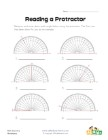## Reading a Protractor Worksheet 2

Read each protractor and write down the angles ...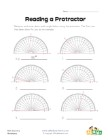## Reading a Protractor Worksheet 1

Read each protractor and write down the angles ...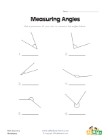## Measuring Angles Worksheet 2

Use a protractor to measure each of the angles.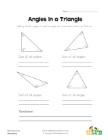## Angles in a Triangle Worksheet

Kids learn that the angles of a triangle always...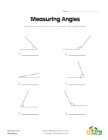## Measuring Angles Worksheet 1

Measure each of the angles with a protractor an...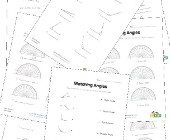## Angles Worksheets

Help kids learn about angles with this collecti...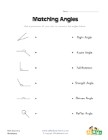## Matching Angles Worksheet

Draw a line to match each of the angles with th...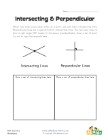## Intersecting and Perpendicular Lines Worksheet

Learn about intersecting and perpendicular lines.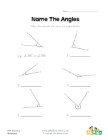## Name the Angles Worksheet

Follow the example and name each of the angles.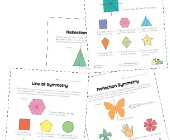## Symmetry Worksheets

Help kids learn about the concept of symmetry w...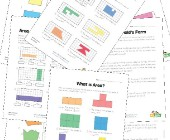## Area and Perimeter Worksheets

Help kids learn to calculate the area and perim...